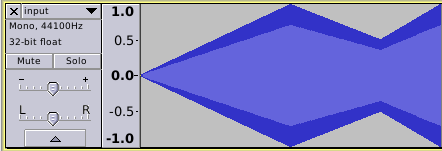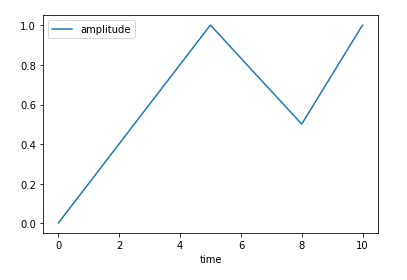# Audio From Scratch With Go: Extracting Breakpoints

So far everything we’ve done with breakpoints involved us creating a breakpoint file and using this to automate part of a track. Now we’ll take a look at how we can take an existing track and extract some breakpoints from this. For this post, we’ll take a look at extracting the amplitudes from the .wave files, as this is one of the most straightforward properties that we can extract.

There is nothing here that we’ve not seen in the previous posts, but rather we are going to combine what we’ve already learned to create a new tool to do this.

In a high level overview, we’ll have to do this:

• iterate over our frames
• record the given amplitude (frame value)
• write to breakpoint file

In essence this would be simple enough, but we’ll make two small modifications to this algorithm. First of all, we don’t want to just generate a breakpoint for each frame, this would just result in a list that is equal to our frame data. We’ll use less space by just taking a snapshot at a certain interval, e.g every 10ms, and take advantage of our linear interpolation that happens when looking up the value of a breakpoint at a certain time.

A small extention to our current breakpoint code is that we need to be able to ‘batch’ the samples into slices of a given time.

If you want to get to the code, everything can be found on GitHub.

# Batching Samples

The BatchSamples function will take a `Wave` and `seconds float64` as input, as this gives us enough data to batch the frames. Given a certain SampleRate, we know how many Samples per Second we are seeing in the file. With this information, we can find the amount of Frames per Seconds. Although frames per second is only equal to the samples per second for mono files, but adapting for multi-channel audio is just a matter of multiplying the SampleSize by the amount of channels.

The SampleSize can thus be expressed as follows: `SampleSize = SampleRate * Channels * seconds`

When we know our sample size, we can split the raw frames into slices of this size.

 `````` 1 2 3 4 5 6 7 8 9 10 11 12 13 14 15 16 17 18 19 20 21 22 23 24 25 26 27 28 29 30 31 `````` ``````func BatchSamples(data Wave, seconds float64) [][]Frame { if seconds == 0 { return [][]Frame{ data.Frames, } } samples := data.Frames sampleSize := int(float64(data.SampleRate * data.NumChannels) * float64(seconds)) batches := len(samples) / sampleSize if len(samples)%sampleSize != 0 { batches++ } batched := make([][]Frame, batches) // this should be round up.. for i := 0; i < len(batched); i++ { start := i * sampleSize if start > len(samples) { return batched } maxTake := i*sampleSize + sampleSize if maxTake >= len(samples)-1 { maxTake = len(samples) } subs := samples[start:maxTake] batched[i] = subs } return batched } ``````

# Extracting data from batches

Once we can split our frames into batches, we can call `BatchSamples(wave,x)` to get the requested batches of a given duration. Then, we can find the max amplitude over all of these and keep track of this over all batches.

The setup code is the same as usual, where we parse an input file with GoAudio. Except that now we’ll also send the frames to the ‘BatchSamples’ function. (Error handling ignored for brevity)

 `````` 1 2 3 4 5 6 7 8 9 10 `````` ``````func main() { flag.Parse() infile := *input outfile := *output wave, _ := wav.ReadWaveFile(infile) frameDuration := 15.0 // 15 ms ticks := frameDuration / 1000.0 batches := wav.BatchSamples(wave, ticks) ... ``````

After doing this, we’ll have our samples batched in 15 ms slices. We’ll continue by iterating over them and collecting the max amplitude found in each. As we’ll need to write this to an output file in the end, we’ll store this in a StringBuilder for now.

One more thing we have to keep track of is which time will be inserted in the `time:value` pair for the breakpoint file. This time value will be a 15ms increment for each batch.

We continue the main function:

 `````` 1 2 3 4 5 6 7 8 9 10 11 12 `````` `````` ... strout := strings.Builder{} elapsed := 0.0 for _, b := range batches { maxa := maxAmp(b) es := strconv.FormatFloat(elapsed, 'f', 8, 64) fs := strconv.FormatFloat(maxa, 'f', 8, 64) strout.WriteString(es + ":" + fs + "\n") elapsed += ticks } ioutil.WriteFile(outfile, []byte(strout.String()), 0644) } ``````

Finally all that is left is to fill our the `maxAmp` function by iterating over each frame in the slice and returning the maximum amp.

 `````` 1 2 3 4 5 6 7 8 9 10 11 12 `````` ``````func maxAmp(ss []wav.Frame) float64 { if len(ss) == 0 { return 0 } max := -1.0 // because they are in range -1 .. 1 for _, a := range ss { if float64(a) > max { max = float64(a) } } return float64(max) } ``````

# Running the breakpoint extractor

When we run this program `go run main.go -i input.wav -w 15 -o output.brk` we can generate a breakpoint file. I’ve created a sample waveform (something I’ll write a future blogpost about) with variable amplitude. You can listen to it here.

The extracted breakpoint file can be seen here.

Using audacity and your favourite plotting tool you can compare the waveform with what the breakpoint extractor generated.# Resources

If you liked this and want to know when I write new posts, the best way to keep up to date is by following me on twitter.